# Insert Mathematical expressions in iBooks

 SEARCH THIS SITE @import url(http://www.google.com/cse/api/branding.css);Custom Search
 You can insert mathematical expressions, equations, functions, etc in iBooks. Click "Insert" then "Equation" at the bottom. A small window pops up where mathematical expressions in LaTex or MathMl format are inserted. In the examples, we shall use LaTex coding. Here is more on LaTex coding.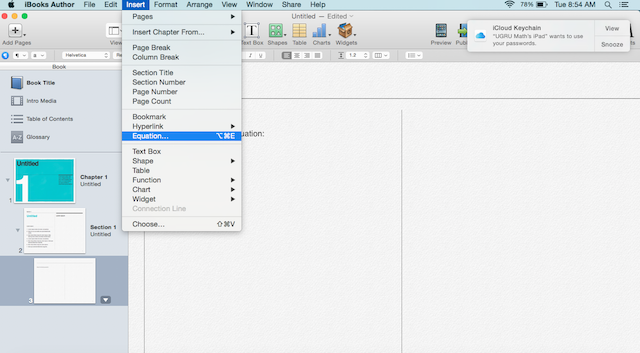. First select the position where you want the equation to be inserted. Example 1: type the equation x^2 + 2x =9 in the window then click "Insert".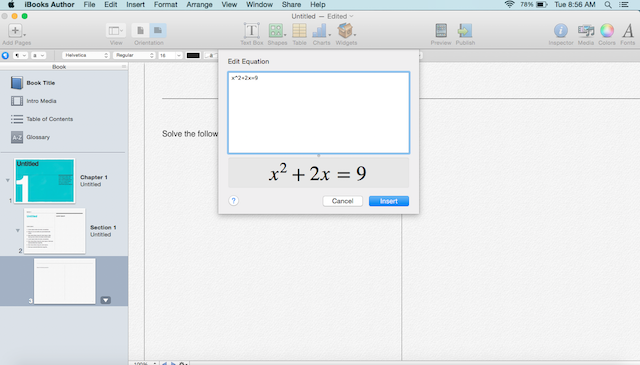. You end up with an equation on the page which in fact may be selected by clicking on it. The position, font color and size and many other properties may now be changed in a similar way as changing properties of letters.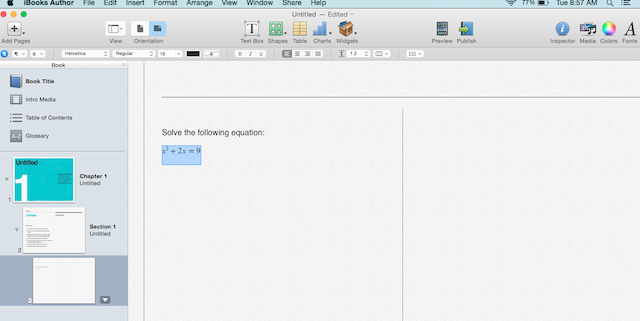. An example with a fraction typed as: \frac{2x+3}{x-1}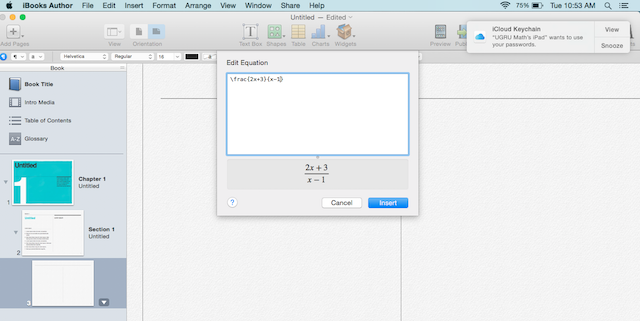. An example with an integral typed as: \int 3x \sin(2x^2-2)dx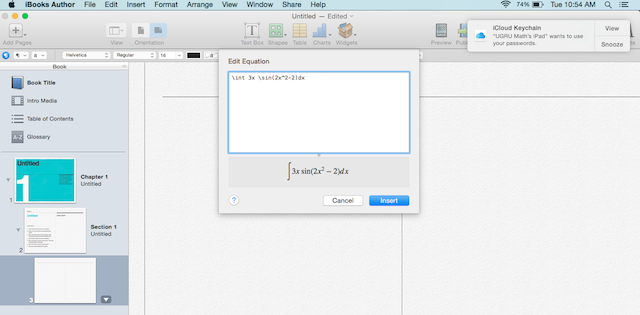. An example with a matrix typed as: \begin{bmatrix} x_1 & x_2 x_3 & x_4 \end{bmatrix}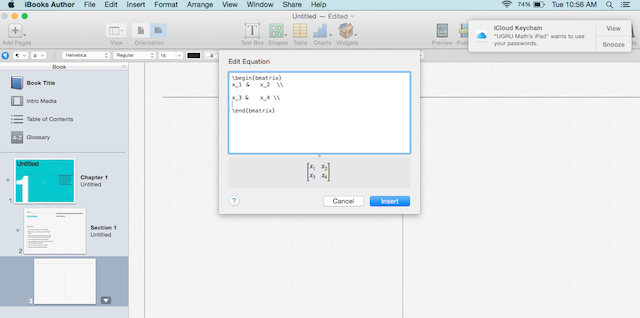. It is possible to also change the color of different part of a mathematical expression in an iBook. The example below was typed as follows \color{red}{(2x-2)} \color{blue}{\log(x+3)}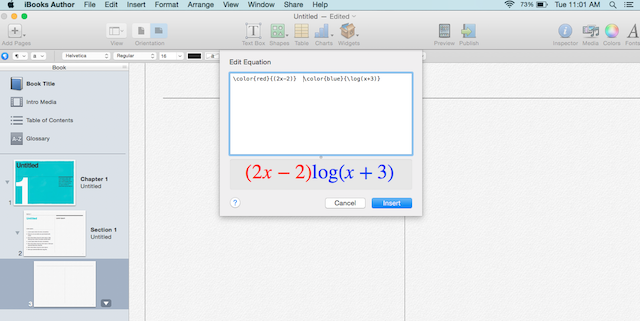. More example and how they are typed are shown below.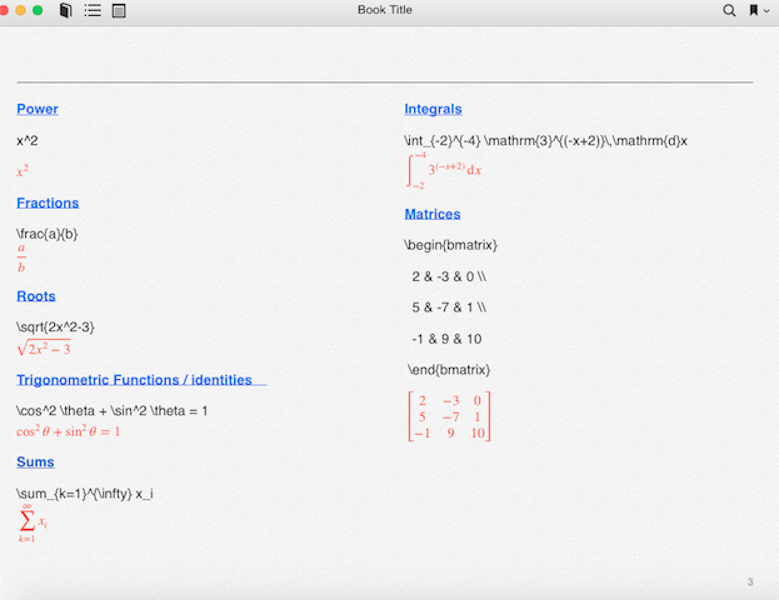.